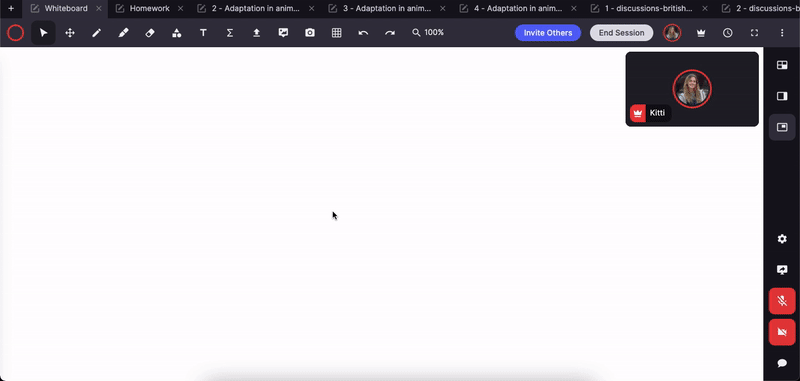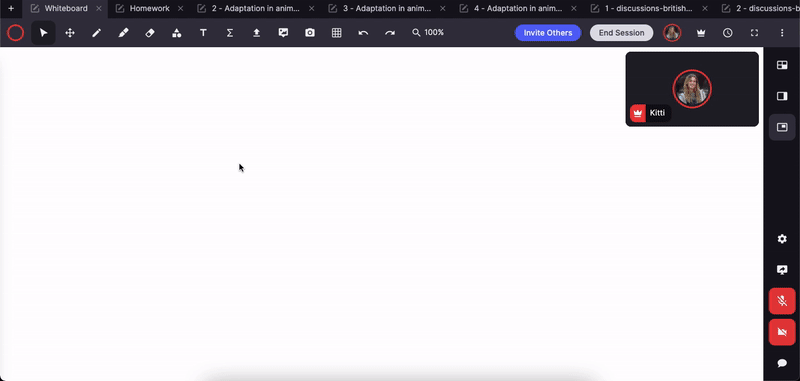# Equation Tool (Formula and Graph editor)

The equation tool is one of the most popular features on Lessonspace and is used by many of our customers tutoring STEM (science, technology, engineering, and maths). There are 2 parts of the equation tool:

1. Formula Mode
2. Graph Mode

Follow the steps below to use the equation tool in your lessons or watch our video tutorial here:

### Formula Mode

Click on the Equation tool and choose Formula. Enter your formula into the Equation editor and hit insert to add it to the Whiteboard. You can ask your students to do the same.  Edit your formula by double-clicking on it or click the Edit Equation button in the floating menu.

Take note of the quick shortcuts: two of the most useful shortcuts are for generating properly formatted fractions and exponents. Simply typing / will put any preceding string of characters as the numerator of a fraction. Similarly, typing ^ (Shift+6 on most QWERTY keyboards) will shift the cursor up into the exponent slot for the preceding string of characters.### Graph Mode

Type in the function you want to plot, adjust your view of the axes, and hit the “Insert Plot” button. The graph will be dropped into your whiteboard (just like an image) and you can move it, resize it and annotate it as you teach.Find out more about our subject-specific tools here and more tools for maths tutoring here.